# NCERT Solutions for Class 4 Maths Halves and Quarters

NCERT solutions for Class 4 Mathematics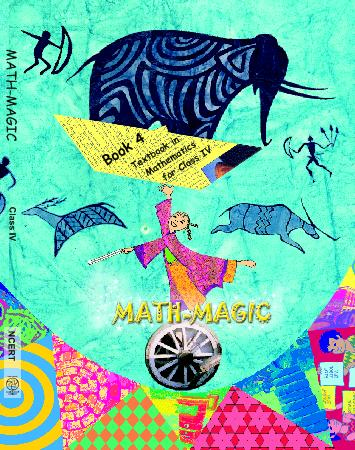## NCERT Solutions for Class 4 Maths Halves and Quarters

Chapter -9 Class 4 Maths Halves and Quarters

### 1. If the cats ask you to divide the chapati equally how will you divide it?

Ans. To divide the chapatti equally, I will fold it in such a way that one part coincides with the other exactly. These parts will look as shown in the figure.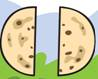### 2. If two or more cats come for food how will you divide one chapati equally for four cats?

Ans. To divide one chapati equally into four parts. I will first divide into two halves. Then. I will fold each half in such a way that one part coincides with the other exactly. These parts will look as under: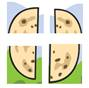### 3. Half of Many Pieces

Rani got a chocolate. She divided it equally and gave half to her friend Reena.

(a) Circle the portion the Reena got.

Ans. (a) Reena’s portion is shown as encircled: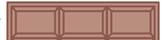(b) How many pieces of chocolate are there?

Ans. (b) There are six pieces of chocolate.

(c) How many pieces were left with Rani?

Ans. (c) Three pieces of chocolate were left with Rani.

### 4. Rajni’s father brought a cake. She divided the cake into 4 equal parts- for herself, her brother  Raju, her father and her mother.

(a) Colour each share with different colours.

Ans.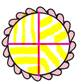(b) How much does each get?

Ans. (b) Each gets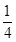of the cake.

(c) Out of 4 parts Rajni will get 2 parts. Which is equal to half of the cake. So she can write as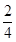or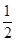.

Ans. (c)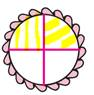(d) Colour the share Raju got.

Ans. (d)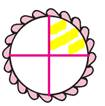### 5. Using a Price List:(a) How much doeskg tomato costs?

Ans. (a) Cost of 1 kg tomato = Rs8

Cost ofkg of tomato = Rs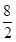= Rs 4

(b) Which costs morekg of onions orkg of carrots.

Ans. (b) Cost of 1 kg of onions = Rs. 10

Cost ofkg of onions = Rs.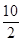= Rs 5

Cost of 1 kg of carrots = Rs 16

Cost ofkg of carrots = Rs.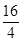= Rs 4

Since, 5 is more than 4, therefore, cost ofkg of onions is more than the cost ofkg of onions.

(c) What is the price of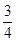kg potato?

Ans. (c) Cost of 1 kg potato = Rs. 12

Cost ofkg of potato = Rs 12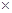= Rs.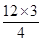= Rs. 9

(d) Keerthi is going for shopping. She has only Rs 20 with her. Can she buy all the things in her shopping list?

Ans. (d) Cost ofkg potato = Rs. 8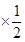= Rs. 4

Cost of 2 kg pumpkin = Rs. 42 = Rs. 8

Cost ofkg carrot = Rs. 16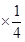= Rs. 4

Total cost = Rs. 16

Since, Keerthi has Rs. 20 which are more than Rs. 16, so she can buy all the things in her shopping list.

### 6. (a) What part of the whole is coloured? Write below each shape.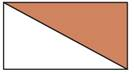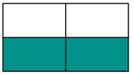Ans.is coloured andoris coloured.

(b) Colour that part of the shapes which is written below.

Ans.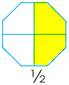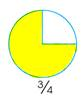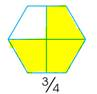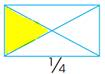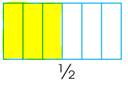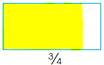### 7. Match the colour part as shown.

Ans.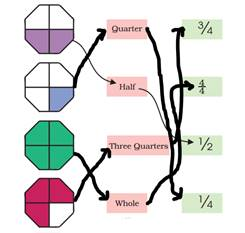### 8. How many centimeters long is the line?

Ans. The line is 50 cm long (because 1 metre = 100 cm)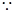1 metre = 100 cmmetre = 50 cm100= 50metre = 25 cm100= 25metre = 75 cm100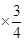= 75

### 9. (a) How many millimetres of milk does each bottle have?

Ans. (a) Each bottle has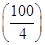= 250 ml of milk.

(b) Shade the level of milk in each bottle.

Ans. (b) The level of milk is shaded as shown in the adjacent figure.

(c) How many milliliter of milk does each bottle hold?

Ans. (c) Milk in the first bottle =1000 ml = 750 ml

Milk in the first bottle =1000 ml = 250 ml

### 10. Choose from the weights above to make the two pans equal. In how many ways you can do it?

Ans. Two pans can be made equal by putting the weights shown in the empty pan in many ways. Some of them are given below:

(i) 1 kg, 500 g, 500 g

(ii) 1 kg, 500 g, 300 g, 200 g

(iii) 1 kg, 500 g, 250 g, 250 g

(iv) 1 kg, 250 g, 250 g, 250 g

(v) 1 kg, 500 g, 200 g, 200 g, 100 g

(vi) 1 kg, 500 g, 250 g, 200 g, 50 g

(vii) 500 g, 500 g, 250 g, 250 g, 250 g, 200 g, 50 g

(viii) 500 g, 500 g, 250 g, 250 g, 200 g, 200 g, 100 g

### 11.(a) Draw the weights in empty pan. Remember 1 kg = 1000g.

Ans. (a) To balance the two pans, we will put one weight of 1 kg and two weights 500 g in the empty pan.

(b) In how many different ways can you balance this weight ofkg?

Ans. (b) Some of weights which can balancekg of weight are as under:

(1) 500 g, 250 g; (2) 250 g, 250 g, 50 g; (3) 250 g, 200 g, 200 g, 100 g.

Class 4 Maths Halves and Quarters

### 12. Kannan shaded some parts as shown. But his friend Mini says that it wrong. Explain why it is wrong.

Ans. In the case of the rectangle, the four divisions are not of the equal size. So, cannot be said the shaded part is. In case of the triangle, the line is not at the middle.

Clearly, the shaded part is not.

### 13. There are 60 mangoes.of them are ripe. How many mangoes are ripe?

Ans. Number of mangoes =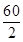= 30.

### 14. There are 32 children.of them are girls. How many children are boys?

Ans. Total number of children = 32

Number of girls =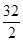= 16

Thus, number of boys = 32- 16= 16.

Class 4 Maths Halves and Quarters

### 15. There are 20 stars. A quarter of them are red. How many stars are red. How many are not red?

Ans. Total number of stars = 20

Number of red stars = A quarter of 20 stars

=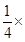20 = 5 stars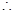Number of non-red stars = 20 – 5 = 15.

### 16. Ravi wants a pencil. It costs Rs. 2. He gives a one-rupee coin, one half rupee coin and one-quarter rupee coin. Is it enough?

Ans. Cost of a pencil = Rs. 2

Money paid = Re 1.00 + Re 0.50 + Re 0.25 = Rs. 1.75

Since, Rs 2 is more than Rs 1.75, therefore, money paid is not enough.

## NCERT solutions for Class 4 Mathematics Chapter 9 Halves and Quarters

Building with BricksView Solutions
Long and ShortView Solutions
A trip to BhopalView Solutions
Tick Tick TickView Solutions
The Way the World LooksView Solutions
The Junk SellerView Solutions
Jugs and MugsView Solutions
Carts and WheelsView Solutions
Halves and QuartersView Solutions
Play With PatternsView Solutions
Table and SharesView Solutions
How Heavy How LightView Solutions
Fields And FencesView Solutions
Smart ChartsView Solutions

NCERT Solutions Class 4 Maths Halves and Quarters PDF (Download) Free from myCBSEguide app and myCBSEguide website. Ncert solution class 4 Maths includes text book solutions from Class 4 Maths Book . NCERT Solutions for CBSE Class  Maths have total 14 chapters. 4 Maths NCERT Solutions in PDF for free Download on our website. Ncert Maths class 4 solutions PDF and Maths ncert class 4 PDF solutions with latest modifications and as per the latest CBSE syllabus are only available in myCBSEguide.

## CBSE app for Students

To download NCERT Solutions for Class 4 Maths, EVS Hindi English, Maths Science do check myCBSEguide app or website. myCBSEguide provides sample papers with solution, test papers for chapter-wise practice, NCERT solutions, NCERT Exemplar solutions, quick revision notes for ready reference, CBSE guess papers and CBSE important question papers. Sample Paper all are made available through the best app for CBSE students and myCBSEguide website.### Test Generator

Create question paper PDF and online tests with your own name & logo in minutes.### myCBSEguide

Question Bank, Mock Tests, Exam Papers, NCERT Solutions, Sample Papers, Notes

### 3 thoughts on “NCERT Solutions for Class 4 Maths Halves and Quarters”

1. Beautiful

2. I have to no problem

3. I can’t understand the problem